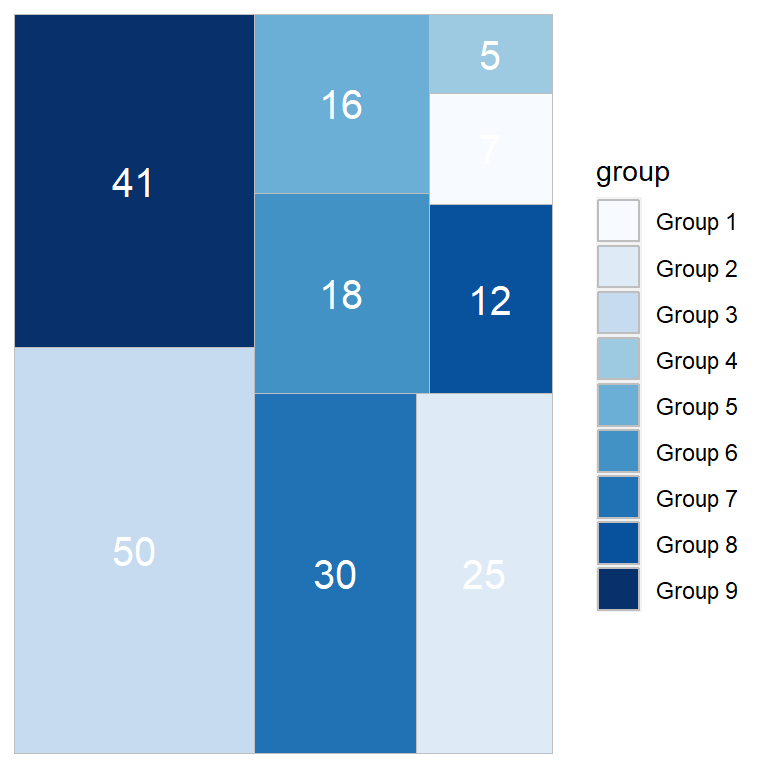# Treemaps in ggplot2 with treemapify

David Wilkins

## Sample data

The following data frame with three columns containing the group and the subgroup of different measures will be used in this tutorial.

``````group <- paste("Group", 1:9)
subgroup <- c("A", "C", "B", "A", "A",
"C", "C", "B", "B")
value <- c(7, 25, 50, 5, 16,
18, 30, 12, 41)

df <- data.frame(group, subgroup, value) ``````

## Basic treemaps with `geom_treemap`

Fill by the categorical variable

The `treemapify` package allows creating treemaps in ggplot2. You will need to specify the variables inside `aes` as follows and use `geom_treemap`.

``````# install.packages("treemapify")
library(treemapify)
# install.packages("ggplot2")
library(ggplot2)

ggplot(df, aes(area = value, fill = group)) +
geom_treemap()``````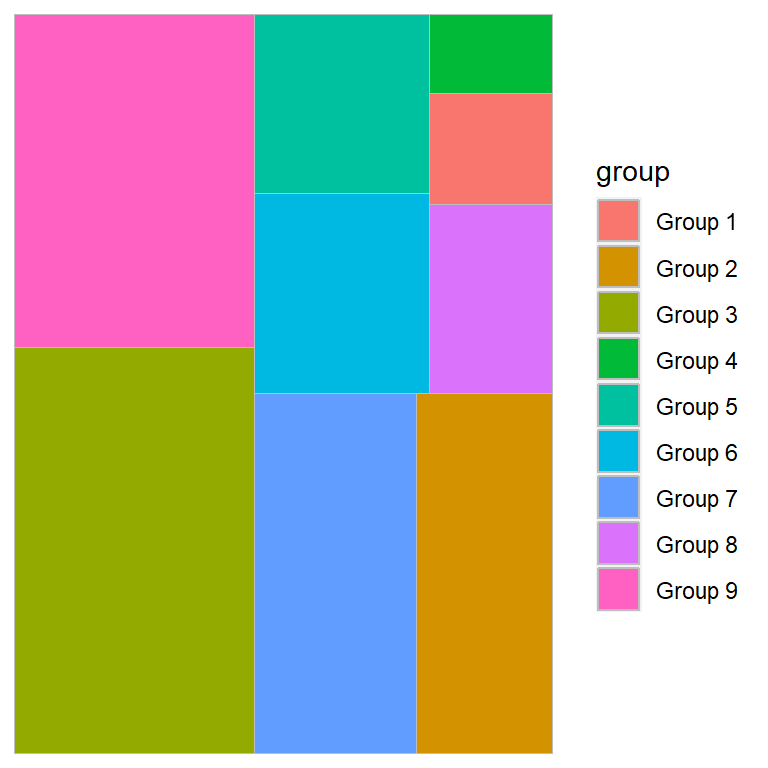Fill by the numerical variable

Note that you can also fill the areas based on the numerical variable depending on what you are trying to represent.

``````# install.packages("treemapify")
library(treemapify)
# install.packages("ggplot2")
library(ggplot2)

ggplot(df, aes(area = value, fill = value)) +
geom_treemap()``````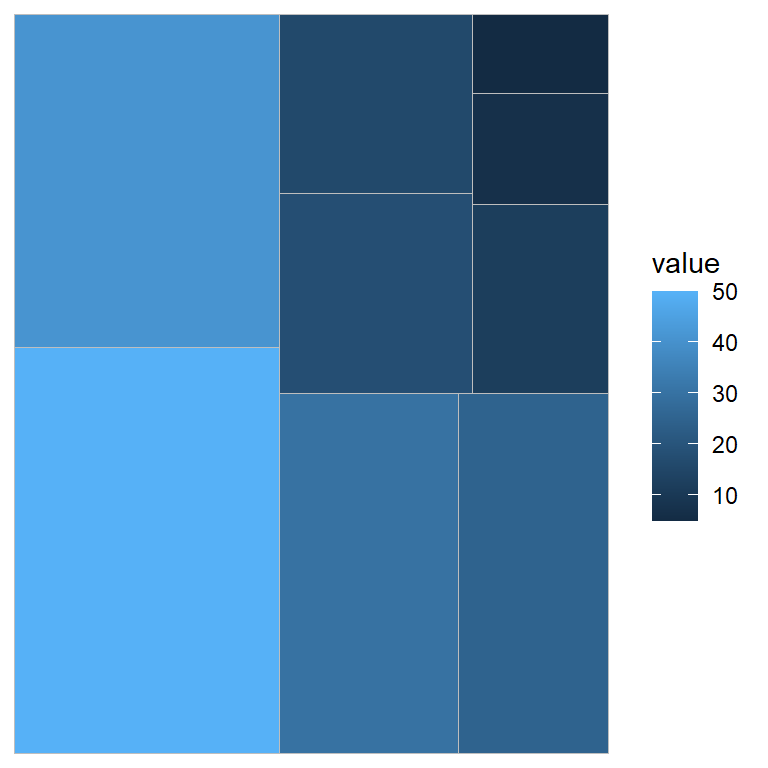## Adding labels to the tiles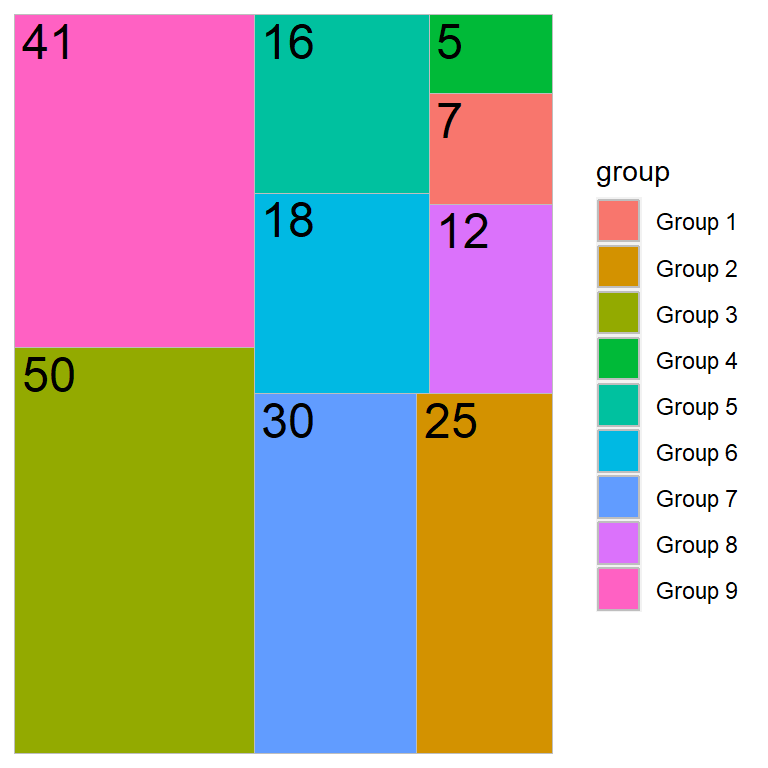Passing a variable to the `label` argument of `aes` and using `geom_treemap_text` you will be able to add labels to each tile of the treemap.

``````# install.packages("treemapify")
library(treemapify)
# install.packages("ggplot2")
library(ggplot2)

ggplot(df, aes(area = value, fill = group, label = value)) +
geom_treemap() +
geom_treemap_text()``````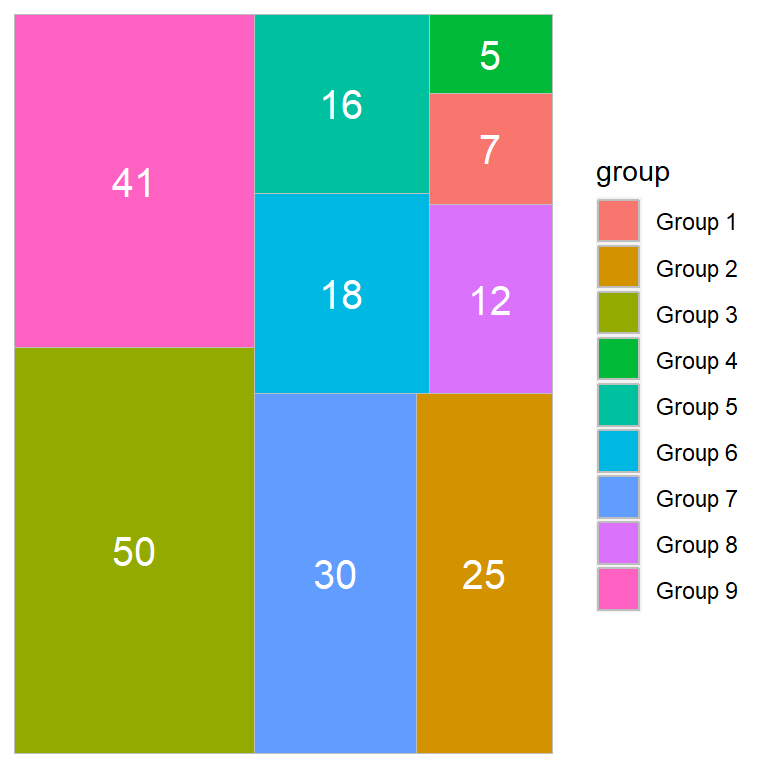The labels can be customized with several arguments. Type `?geom_treemap_text` for the full list.

``````# install.packages("treemapify")
library(treemapify)
# install.packages("ggplot2")
library(ggplot2)

ggplot(df, aes(area = value, fill = group, label = value)) +
geom_treemap() +
geom_treemap_text(colour = "white",
place = "centre",
size = 15)``````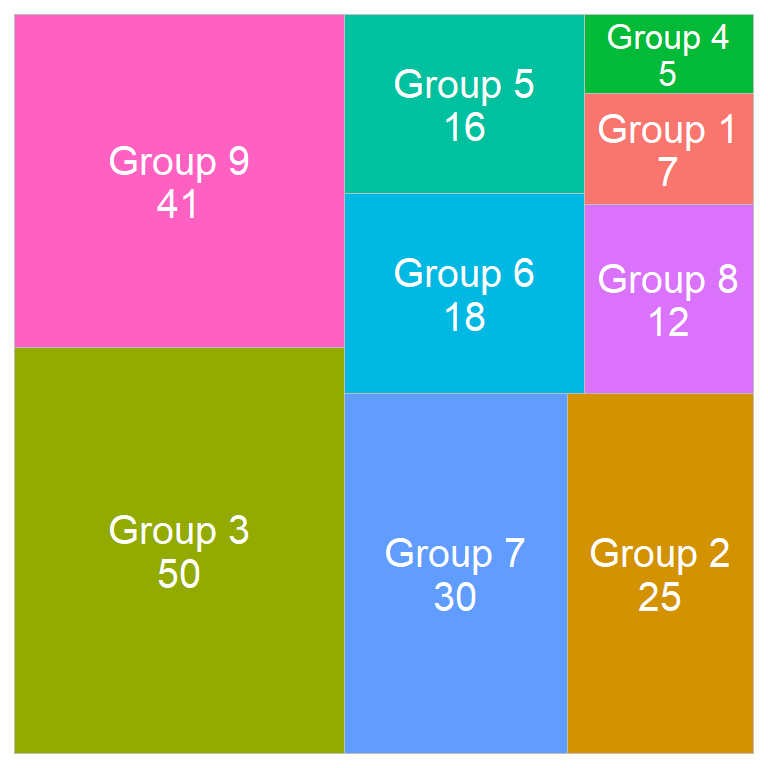You can also add the labels and the groups at the same time and remove the legend if needed.

``````# install.packages("treemapify")
library(treemapify)
# install.packages("ggplot2")
library(ggplot2)

ggplot(df, aes(area = value, fill = group,
label = paste(group, value, sep = "\n"))) +
geom_treemap() +
geom_treemap_text(colour = "white",
place = "centre",
size = 15) +
theme(legend.position = "none")``````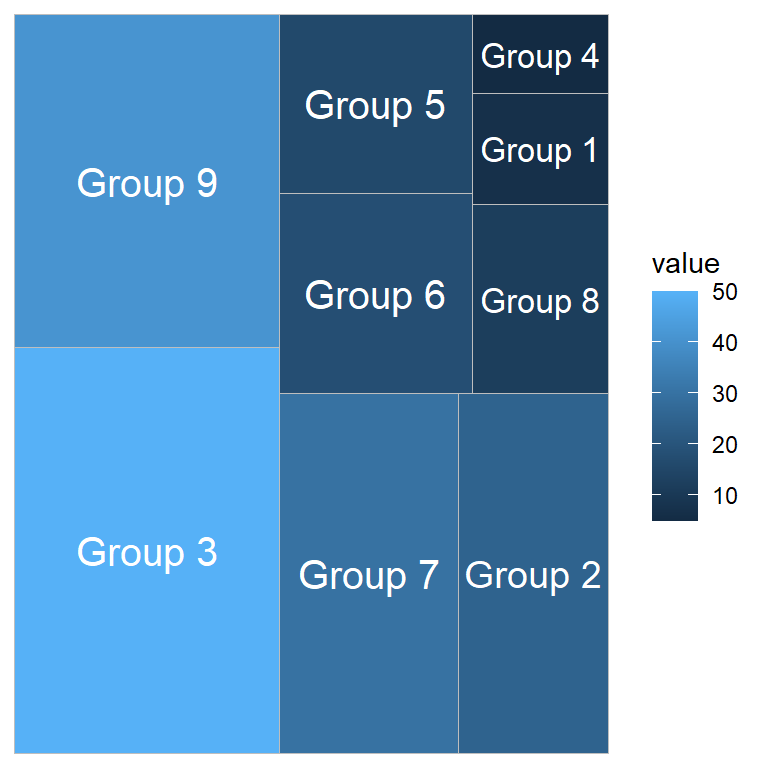If you passed the numerical variable to `fill` you can pass the groups to `label`.

``````# install.packages("treemapify")
library(treemapify)
# install.packages("ggplot2")
library(ggplot2)

ggplot(df, aes(area = value, fill = value, label = group)) +
geom_treemap() +
geom_treemap_text(colour = "white",
place = "centre",
size = 15)``````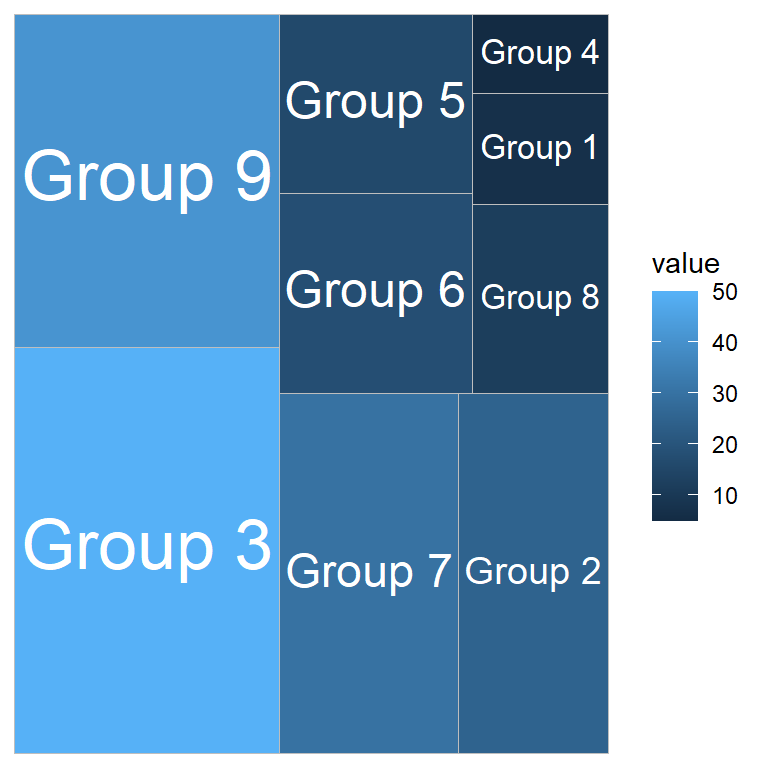Note that there is a logical argument named `grow` that can be set to `TRUE` to fit the text to the tiles.

``````# install.packages("treemapify")
library(treemapify)
# install.packages("ggplot2")
library(ggplot2)

ggplot(df, aes(area = value, fill = value, label = group)) +
geom_treemap() +
geom_treemap_text(colour = "white",
place = "centre",
size = 15,
grow = TRUE)``````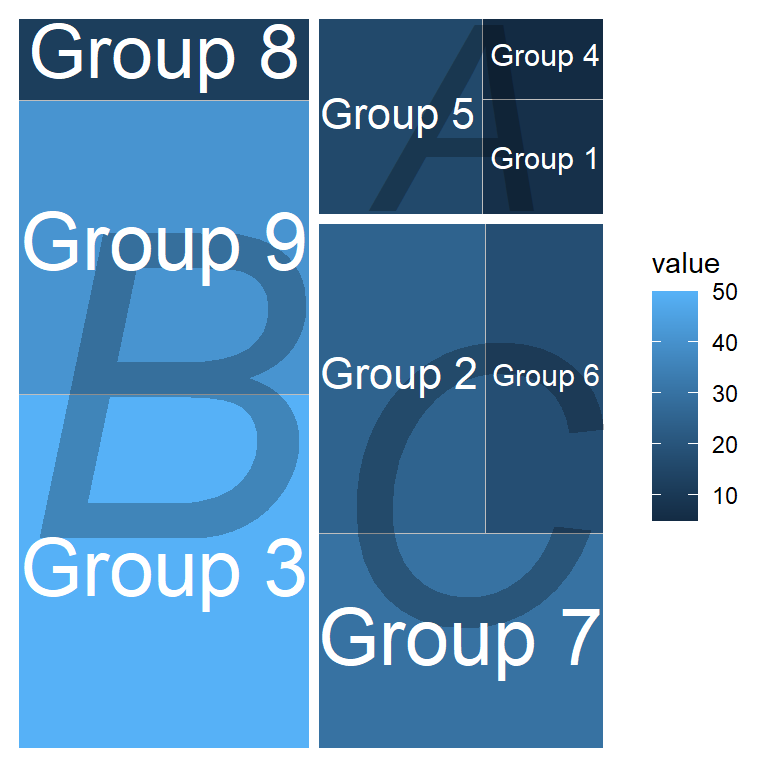If your data set contains a column representing subgroups you can add them to the plot with `subgroup` and `geom_treemap_subgroup_text`. The border of the subgroups can be modified with `geom_treemap_subgroup_border`.

``````# install.packages("treemapify")
library(treemapify)
# install.packages("ggplot2")
library(ggplot2)

ggplot(df, aes(area = value, fill = value,
label = group, subgroup = subgroup)) +
geom_treemap() +
geom_treemap_subgroup_border(colour = "white", size = 5) +
geom_treemap_subgroup_text(place = "centre", grow = TRUE,
alpha = 0.25, colour = "black",
fontface = "italic") +
geom_treemap_text(colour = "white", place = "centre",
size = 15, grow = TRUE)``````

You can add up to three subgroups with `subgroup2`, `geom_treemap_subgroup2_border` and `geom_treemap_subgroup2_text` and `subgroup3`, `geom_treemap_subgroup3_border` and `geom_treemap_subgroup3_text`.

## Color customization

The colors of the treemap can be customized with a color scale. The choice will depend on the data you pass to `fill` (continuous or discrete). You can check a couple of examples below.

Continuous

``````# install.packages("treemapify")
library(treemapify)
# install.packages("ggplot2")
library(ggplot2)

ggplot(df, aes(area = value, fill = value, label = group)) +
geom_treemap() +
geom_treemap_text(colour = c(rep("white", 2),
1, rep("white", 6)),
place = "centre", size = 15) +
scale_fill_viridis_c()``````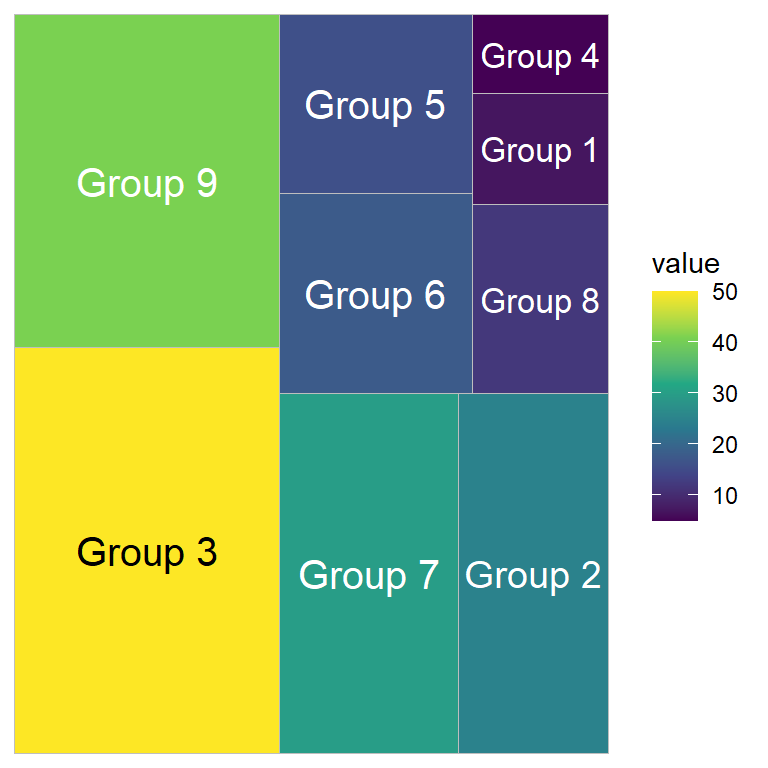Discrete

``````# install.packages("treemapify")
library(treemapify)
# install.packages("ggplot2")
library(ggplot2)

ggplot(df, aes(area = value, fill = group, label = value)) +
geom_treemap() +
geom_treemap_text(colour = "white",
place = "centre",
size = 15) +
scale_fill_brewer(palette = "Blues")``````